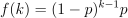/NumPy 1.14

# numpy.random.geometric

`numpy.random.geometric(p, size=None)`

Draw samples from the geometric distribution.

Bernoulli trials are experiments with one of two outcomes: success or failure (an example of such an experiment is flipping a coin). The geometric distribution models the number of trials that must be run in order to achieve success. It is therefore supported on the positive integers, `k = 1, 2, ...`.

The probability mass function of the geometric distribution iswhere `p` is the probability of success of an individual trial.

Parameters: p : float or array_like of floats The probability of success of an individual trial. size : int or tuple of ints, optional Output shape. If the given shape is, e.g., `(m, n, k)`, then `m * n * k` samples are drawn. If size is `None` (default), a single value is returned if `p` is a scalar. Otherwise, `np.array(p).size` samples are drawn. out : ndarray or scalar Drawn samples from the parameterized geometric distribution.

#### Examples

Draw ten thousand values from the geometric distribution, with the probability of an individual success equal to 0.35:

```>>> z = np.random.geometric(p=0.35, size=10000)
```

How many trials succeeded after a single run?

```>>> (z == 1).sum() / 10000.
0.34889999999999999 #random
```